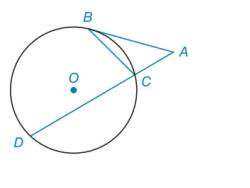Chapter 6.2, Problem 23EElementary Geometry For College St...

7th Edition
Alexander + 2 others
ISBN: 9781337614085

Solutions

Chapter
SectionElementary Geometry For College St...

7th Edition
Alexander + 2 others
ISBN: 9781337614085
Textbook Problem

In Exercises 23 to 25 , complete a paragraph proof. Given: Tangent A B ¯ to ⊙ ο at point B m ∠ A = m ∠ B Prove: m B D ⌢ = 2 ⋅ m B C ⌢To determine

To find:

The prove mBD=2mBC.

Explanation

Calculation:

Given,

mA=mB.

To prove: mBD=2mBC.

Since, Tangent AB¯ to ο at point B.

A is a tangent-secant angle, mA=mBDmBC2.

B has the vertex on the circle and is a chord-tangent angle, mB=mBC2.

Given,

mA=mB

Substitute the values of mA and mB in mA=mB

Still sussing out bartleby?

Check out a sample textbook solution.

See a sample solution

The Solution to Your Study Problems

Bartleby provides explanations to thousands of textbook problems written by our experts, many with advanced degrees!

Get Started

For the following scores, find the value of each expression: X 3 2 4 2 a. X b. (X)2 c. X 2 d. (X 2)

Essentials of Statistics for The Behavioral Sciences (MindTap Course List)